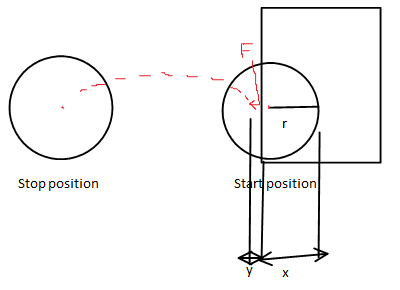# What is the distance traveled

A rough sketch of experiment.the red dots represent a side view of path traveled, F is downward force and the tool used here is a pen placing parallel to the coin

Hi. I have newly started to study mechanical physics. based on study, I conduct a simple experiment. But unfortunately i am unable apply the laws in reality.

Experiment:
I placed a coin(2 Indian Rupee coin) with radius "r" positioned flat part of coin parallel to base of my laptop. Also placed at edge of base such that "x mm" of diameter of coin is supported by laptop while 2r-x is free, unsupported with the coin in a balanced position. Now at "x+y mm < r" measured from center of coin toward the free end I applied a random force perpendicular to laptop base.

Now my question is , how can we compute(formulate) distance "z" traveled by coin measured from center of coin at start point to center of coin at the place it stopped in terms of known variables mentioned below.

My attempt:
To me the known values are : r,x,y,density of coin(p),width(or height) of coin(w),time it took to stop(t).
Here for simplicity I have not considered the torque generated, and visualized the traveled path as a simple parabolic path. Now I can measure the distance traveled
z = v0t+(1/2)gt2. where g is acceleration due to gravity.

But how can I find the initial velocity v0. I know v0 is not zero, since the situation here is not equivalent to a freely falling scenario.

Last edited:

## Answers and Replies

Is the force acting up or down?

Is the force acting up or down?

It's a downward force.

tiny-tim
Science Advisor
Homework Helper
hi smslca!assuming the coin doesn't slip, it will rotate around the edge of the laptop until the reaction force is zero

calculate the velocity (magnitude and direction) of the centre of mass at that instant: that is your vo and θ(but it probably will slip, and if the edge is curved not sharp then that makes the maths even more difficult)